Function Repository Resource:

# StandardOrderIndex

Get the index of a list of integers in standard order

Contributed by: Ed Pegg Jr
 ResourceFunction["StandardOrderIndex"][list] gives the index of a list of integers in standard order.

## Details and Options

Integers >0 in standard order start with 1, then can never be more than 1 higher than all previous integers.
The index is acquired by first noting the positions of the highest numbers to make a binary number.
The table below illustrates the process for determining an index for a standard order:
 {1,2,2,1,3,2,1,4,3} integers >0 in standard order start with 1, then can never be more than 1 higher than all previous integers. {1,2,0,0,3,0,0,4,0} some of the digits are new highest digits. {0,0,2,1,0,2,1,0,3} other digits acquire a mixed radix based on the previous highest digit. {1,0,0,1,0,0,1,0} without the initial 1, the sequence of highest digits mapped to 1 values makes a binary number B. 223241 any binary number with 1's→{2,…,n} and sequential zeros acting as powers gives a Bell index. BellB[n] equals the sum of Bell indices of length n-1 binary numbers. {146, 86}->5867 the total of the first B Bell indices plus the mixed radix value gives the index.
The input follows the "standard order" described in the OEIS.

## Examples

### Basic Examples (2)

Find the index of a standard order:

 In:=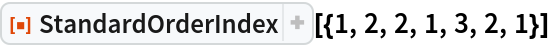Out=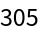Index of a larger standard order:

 In:=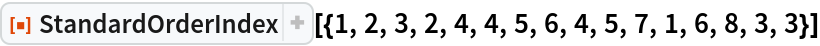Out=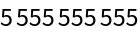### Scope (4)

Define a Bell indexing function:

 In:=The total number of standard orders of length n is given by BellB[n] or the total of the Bell indices with the last standard order being Range[n]:

 In:=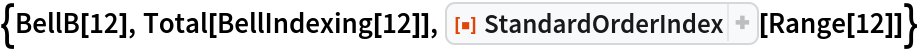Out=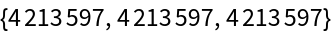If a list isn’t in standard order it will be put into standard order before the indexing. Here is a random list:

 In:=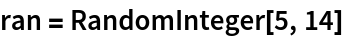Out=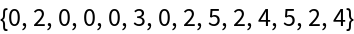Here is its index:

 In:=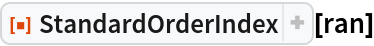Out=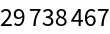Define a standard ordering function:

 In:=Order the random list:

 In:=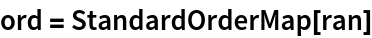Out=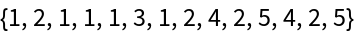Compare the index of the unordered version with that of the ordered one:

 In:=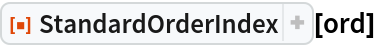Out=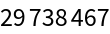Some auxiliary functions:

 In:=Here are the 52 standard orders of length 5:

 In:=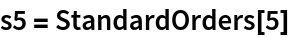Out=Give the indices of these standard orders:

 In:=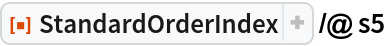Out=A particular standard order:

 In:=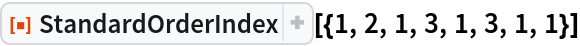Out=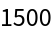Recover that data using the StandardOrders function:

 In:=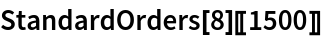Out=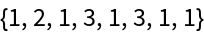Find the index of a standard order:

 In:=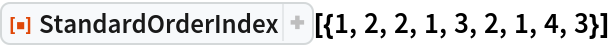Out=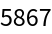Define a Bell indexing function:

 In:=Here is how the function finds the index:

 In:=Out=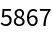### Possible Issues (2)

For length n, 2n indices need to be generated, so this function will start slowing down at length 20:

 In:=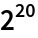Out=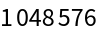There are lots of standard orders of length 20:

 In:=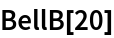Out=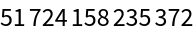## Version History

• 1.0.0 – 31 December 2019